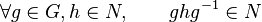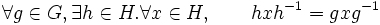First-order subgroup property

Definition

Symbol-free definition

A subgroup property is said to be a first-order subgroup property if it can be expressed using a first-order formula, viz a formula that allows:

• Logical operations (conjunction, disjunction, negation, and conditionals)
• Equality testing
• Quantification over elements of the group and subgroup (this in particular allows one to test membership of an element of the group, in the subgroup)
• Group operations (multiplication, inversion and the identity element)

Things that are not allowed are quantification over other subgroups, quantification over automorphisms, and quantification over supergroups.

Importance

First-order language is severely constricted, at least when it comes to subgroup properties. Hence, not only are there very few first-order subgroup properties of interest, also, very few of the subgroup property operators preserve the first-order nature.

Examples

Normality

Normality is a first-order subgroup property as can be seen from the following definition: a subgroup$N$ of a group$G$ is termed normal if the following holds:$\forall g \in G,h \in N, \qquad ghg^{-1} \in N$

The formula is universal of quantifier rank 1.

Centrality

A subgroup is a central subgroup if it lies inside the center, or equivalently, if every element in the subgroup commutes with every element in the group.

Clearly, the property of being a central subgroup is first-order.

The formula is universal of quantifier rank 1.

Central factor

A subgroup is a central factor if the inner automorphism of the subgroup induced by any element of the group can also be mimicked by an element of the subgroup. This can naturally be expressed as a first-order formula of quantifier rank 3 with the outermost layer being universal.$\forall g \in G, \exists h \in H . \forall x \in H, \qquad hxh^{-1} = gxg^{-1}$

Relation with formalisms

Function restriction formalism

The general question of interest: given a subgroup property with a function restriction expression$a \to b$, can we use the expression to give a first-order definition for the subgroup property? It turns out that the following suffice:

•$a$ should be a first-order enumerable function property (this condition is much stronger than just being a first-order function property because we are not allowed to directly quantify over functions.
•$b$ should be a first-order function property in the sense that given any function, it must be possible to give a first-order formula that outputs whether or not the function satisfies$b$.

The primary example of a first-order enumerable function property is the property of being an inner automorphism. Most function properties that we commonly enoucnter are first-order (that is, they can be tested/verified using first-order formulae).